# I Learn TensorFlow Fundamentals in 21,9 minutesLearn TensorFlow Fundamentals in 20 Minutes. If you were to execute the above in a Python script or shell, it would run, but it wouldn't actually do anything.

### Introduction

TensorFlow is an open source software library created by Google that is used to implement machine learning and deep learning systems.

These two names contain a series of powerful algorithms that share a common challenge — to allow a computer to learn how to automatically spot complex patterns and/or to make best possible decisions.

#### Easily scale code-sharing to build better software faster

Bit plays with Git and NPM to help your team scale code-sharing and build better modular software faster together. Shared components are building blocks, so you can use them across projects and apps. Give it a try:

### TensorFlow: a modern machine learning library

TensorFlow, open sourced to the public by Google in November 2015, is the result of years of lessons learned from creating and using its predecessor, DistBelief.

It was made to be flexible, efficient, extensible, and portable. Computers of any shape and size can run it, from smartphones all the way up to huge computing clusters. It comes with lightweight software that can instantly productionize your trained model, effectively eliminating the need to reimplement models.

TensorFlow embraces the innovation and community engagement of open source, but has the support, guidance, and stability of a large

corporation.

Because of its multitude of strengths, TensorFlow is appropriate for individuals and businesses ranging from startups to companies as large as, well, Google. Since its open source release in November 2015, TensorFlow has become one of the most exciting machine learning libraries available. It is being used more and more in research, production, and education.

The library has seen continual improvements, additions, and optimizations, and the TensorFlow community has grown dramatically.

### TensorFlow: What’s in a Name?

Tensors are the standard way of representing data in deep learning. Simply put, tensors are just multidimensional arrays, an extension of two-dimensional tables (matrices) to data with higher dimensionality.

A tensor, put simply, is an n-dimensional matrix.

In general, you can think about tensors the same way you would matrices, if you are more comfortable with matrix math!

In this article, we will discuss and briefly go through TensorFlows basics: Variables, Sessions, Placeholders, etc Also, we will see how to install the TenorFlow library in your System.

### Installing TensorFlow

If you are using a clean Python installation (probably set up for the purpose of learning TensorFlow), you can get started with the simple pip installation:

```pip install tensorflow
```

This approach does, however, have the drawback that TensorFlow will override existing packages and install specific versions to satisfy dependencies.

If you are using this Python installation for other purposes as well, this will not do. One common way around this is to install TensorFlow in a virtual environment, managed by a utility called `virtualenv`.

Depending on your setup, you may or may not need to install `virtualenv` on your machine. To install `virtualenv`, type:

```pip install virtualenv
```

See http://virtualenv.pypa.io for further instructions.

In order to install TensorFlow in a virtual environment, you must first create the virtual environment — in this book we choose to place these in the ~/envs folder, but feel free to put them anywhere you prefer:

```cd ~
mkdir envs
virtualenv ~/envs/tensorflow
```

This will create a virtual environment named tensorflow in `~/envs` (which will manifest as the folder ~/envs/tensorflow). To activate the environment, use:

```source ~/envs/tensorflow/bin/activate
```

The prompt should now change to indicate the activated environment:

```(tensorflow)
```

At this point the pip install command:

```(tensorflow) pip install tensorflow
```

will install TensorFlow into the virtual environment, without impacting other packages installed on your machine. Finally, in order to exit the virtual environment, you type:

```(tensorflow) deactivate
```

at which point you should get back the regular prompt:

Up until recently TensorFlow had been notoriously difficult to use with Windows machines. As of TensorFlow 0.12, however, Windows

integration is here! It is as simple as:

```pip install tensorflow
```

for the CPU version, or:

```pip install tensorflow-gpu
```

for the GPU-enabled version (assuming you already have CUDA 8).

### “HelloWorld” in TensorFlow

Now, we are done with installing and setting up our TensorFlow environment. Let’s make a simple TensorFlow program that will combine the words “Hello” and “World” and display the phrase — “HelloWorld”.

While simple and straightforward, this example introduces many of the core elements of TensorFlow and the ways in which it is different from a regular Python program.

First, we run a simple install and version check (if you used the `virtualenv`installation option, make sure to activate it before running TensorFlow code):

```/** inst_check.py **/
import tensorflow as tf
print(tf.__version__)
```

The above prints the version of the tensorflow in the terminal. Run the following command to execute the script:

```python inst_check.py
```

```python inst_check.py
1.4.0
```

If correct, the output will be the version of TensorFlow you have installed on your system. Version mismatches are the most probable cause of issues down the line.

We are done with verifying the TensorFlow version. Let’s implement the HelloWorld example. Below is the full code:

```/** helloworld.py **/
import tensorflow as tf
h = tf.constant("Hello")
w = tf.constant(" World!")
hw = h + w
with tf.Session() as sess:
ans = sess.run(hw)
print(ans)
```

We assume you are familiar with Python and imports, in which case the first line:

```import tensorflow as tf
```

requires no explanation. Next, we define the constants “Hello” and “ World!”, and combine them:

```import tensorflow as tf
h = tf.constant(“Hello”)
w = tf.constant(“ World!”)
hw = h + w
```

At this point, you might wonder how (if at all) this is different from the simple Python code for doing this:

```ph = “Hello”
pw = “ World!”
phw = h + w
```

The key point here is what the variable `hw` contains in each case. We can check this using the print

command. In the pure Python case we get this:

```>print phw
Hello World!
```

In the TensorFlow case, however, the output is completely different:

```>print hw
```

Probably not what you expected!

```hw = h + w
```

The TensorFlow line of code does not compute the sum of h and w, but rather adds the summation operation to a graph of computations

to be done later.

Next, the Session object acts as an interface to the external TensorFlow computation mechanism, and allows us to run parts of the computation graph we have already defined. The line:

```ans = sess.run(hw)
```

actually computes hw (as the sum of h and w, the way it was defined previously), following which the printing of ans displays the expected `“Hello World!”` message.

This completes the first TensorFlow example. Run the following command to execute the script:

```python helloworld.py
```

### Session

Following the above code, you have noticed the constant use of Session. It is time we get to know what Session is.

A Session object is the part of the TensorFlow API that communicates between Python objects and data on our end, and the actual computational system where memory is allocated for the objects we define, intermediate variables are stored, and finally results are fetched for us.

```sess = tf.Session()
```

The execution itself is then done with the .run() method of the Session object. When called, this method completes one set of computations in our graph in the following manner: it starts at the requested output(s) and then works backward, computing nodes that must be executed according to the set of dependencies. Therefore, the part of the graph that will be computed depends on our output query.

In our example, we requested that node f be computed and got its value, 5, as output:

```outs = sess.run(f)
```

When our computation task is completed, it is good practice to close the session using the sess.close() command, making sure the resources used by our session are freed up. This is an important practice to maintain even though we are not obligated to do so for things to work:

```sess.close()
```

### A note on constructors

The `tf.<operator>` function could be thought of as a constructor, but to be more precise, this is actually not a constructor at all, but rather a factory method that sometimes does quite a bit more than just creating the operator objects.

### Introduction to Computation Graphs

TensorFlow allows us to implement machine learning algorithms by creating and computing operations that interact with one another. These interactions form what we call a “computation graph,” with which we can intuitively represent complicated functional architectures.

For those to whom this concept is new, a graph refers to a set of interconnected entities, commonly called nodes or vertices. These nodes are connected to each other via edges. In a dataflow graph, the edges allow data to “flow” from one node to another in a directed manner.

In TensorFlow, each of the graph’s nodes represents an operation, possibly applied to some input, and can generate an output that is passed on to other nodes.

Operations in the graph include all kinds of functions, from simple arithmetic ones such as subtraction and multiplication to more complex ones, as we will see later on. They also include more general operations like the creation of summaries, generating constant values, and more.

Let’s take a look at a bare-bones example.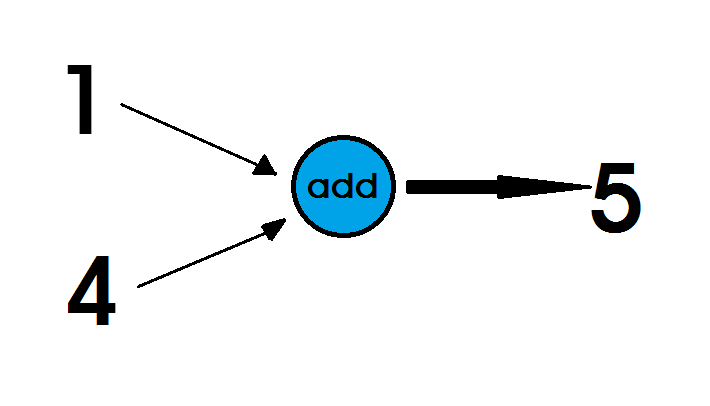In the above example, we see the graph for basic addition. The function, represented by a circle, takes in two inputs, represented as arrows pointing into the function. It outputs the result of adding 1 and 4 together: 5, which is shown as an arrow pointing out of the function. The result could then be passed along to another function, or it might simply be returned to the client. We can also look at this graph as a simple equation: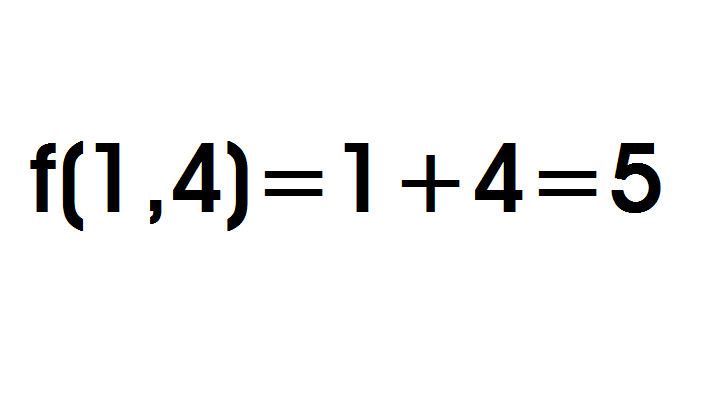The above illustrates how the two fundamental building blocks of graphs, nodes and edges, are used when constructing a computation graph. Let’s go over their properties:

• Nodes, typically drawn as circles, ovals, or boxes, represent some sort of
• computation or action being done on or with data in the graph’s context. In the above example, the operation “add” is the sole node.
• Edges are the actual values that get passed to and from Operations, and are typically drawn as arrows. In the “add” example, the inputs 1 and 2 are both edges leading into the node, while the output 3 is an edge leading out of the node. Conceptually, we can think of edges as the link between different Operations as they carry information from one node to the next.

Now, here’s a slightly more interesting example: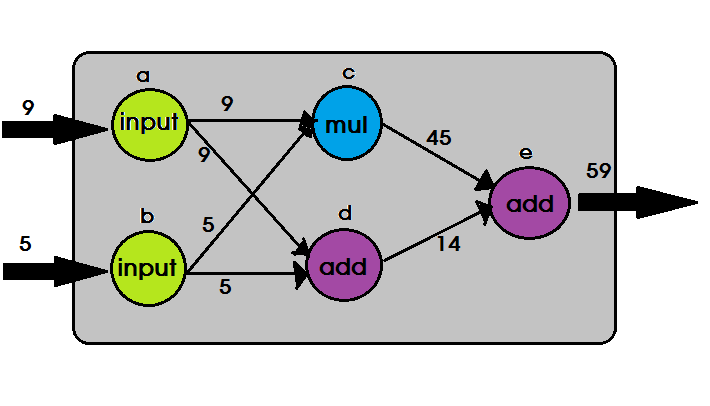There’s a bit more going on in this graph! The data is traveling from left to right (as indicated by the direction of the arrows), so let’s break down the graph, starting from the left.

• 1. At the very beginning, we can see two values flowing into the graph, 9 and 5. They may be coming from a different graph, being read in from a file, or entered directly by the client.
• 2. Each of these initial values is passed to one of two explicit “input” nodes, labeled a and b in the graphic. The “input” nodes simply pass on values given to them- node a receives the value 9 and outputs that same number to nodes c and d, while node b performs the same action with the value 5.
• 3. Node c is a multiplication operation. It takes in the values 9 and 5 from nodes a and b, respectively, and outputs its result of 45 to node e. Meanwhile, node d performs addition with the same input values and passes the computed value of 14 along to node e.
• 4. Finally, node e, the final node in our graph, is another “add” node. It receives the values of 45 and 14, adds them together, and spits out 59 as the final result of our graph.

Here’s how the above graphical representation might look as a series of equations: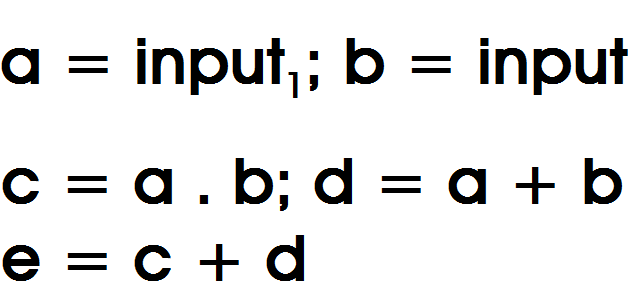If we wanted to solve for e and a=5 and b = 3 , we can just work backwards from e and plug in!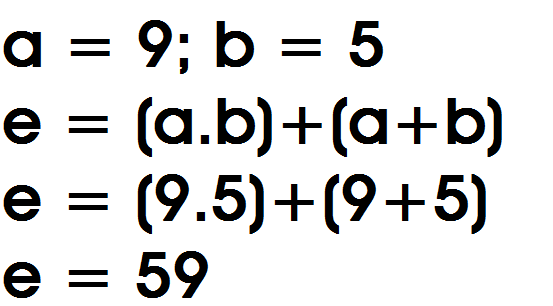With that, the computation is complete! There are concepts worth pointing out here:

• The pattern of using “input” nodes is useful, as it allows us to relay a single input value to a huge amount of future nodes. If we didn’t do this, the client (or whoever passed in the initial values) would have to explicitly pass each input value to multiple nodes in our graph. This way, the client only has to worry about passing in the appropriate values once and any repeated use of those inputs is abstracted away. We’ll touch a little more on abstracting graphs shortly.
• Pop quiz: which node will run first- the multiplication node c, or the addition node d?
• The answer: you can’t tell. From just this graph, it’s impossible to know which of c and d will execute first. Some might read the graph from left-to-right and top-to bottom and simply assume that node c would run first, but it’s important to note that the graph could have easily been drawn with d on top of c. Others may think of these nodes as running concurrently, but that may not always be the case, due to various implementation details or hardware limitations. In reality, it’s best to think of them as running independently of one another. Because node c doesn’t rely on any information from node d, it doesn’t have to wait for node d to do anything in order to complete its operation. The converse is also true: node d doesn’t need any information from node c.

### Building your first TensorFlow graph

We became pretty familiar with the following graph in the last section:

Here’s what it looks like in TensorFlow code:

```import tensorflow as tf
a = tf.constant(9, name=”input_a”)
b = tf.constant(5, name=”input_b”)
c = tf.mul(a,b, name=”mul_c”)
```

Let’s break this code down line by line. First, you’ll notice this import statement:

```import tensorflow as tf
```

This, unsurprisingly, imports the TensorFlow library and gives it an alias of tf. This is by convention, as it’s much easer to type “tf,” rather than “tensorflow” over and over as we use its various functions!

Next, let’s focus on our first two variable assignments:

```a = tf.constant(9, name=”input_a”)
b = tf.constant(5, name=”input_b”)
```

Here, we’re defining our “input” nodes, a and b. These lines use our first TensorFlow Operation: tf.constant(). In TensorFlow, any computation node in the graph is called an Operation, or Op for short. Ops take in zero or more Tensor objects as input and output zero or more Tensor objects.

To create an Operation, you call its associated Python constructor- in this case, tf.constant() creates a “constant” Op. It takes in a single tensor value, and outputs that same value to nodes that are directly connected to it.

For convenience, the function automatically converts the scalar numbers 9 and 5 into Tensor objects for us. We also pass in an optional string name parameter, which we can use to give an identifier to the nodes we create.

```c = tf.mul(a,b, name=”mul_c”)
```

Here, we are defining the next two nodes in our graph, and they both use the nodes we defined previously. Node c uses the tf.mul. Op, which takes in two inputs and outputs the result of multiplying them together.

Similarly, node d uses tf.add, an Operation that outputs the result of adding two inputs together. We again pass in a name to both of these Ops (it’s something you’ll be seeing a lot of).

Notice that we don’t have to define the edges of the graph separately from the node- when you create a node in TensorFlow, you include all of the inputs that the Operation needs to compute, and the software draws the connections for you.

```e = tf.add(c,d, name=”add_e”)
```

This last line defines the final node in our graph. e uses tf.add in a similar fashion to node d. However, this time it takes nodes c and d as input- exactly as its described in the graph above. With that, our first, albeit small, graph has been fully defined! If you were to execute the above in a Python script or shell, it would run, but it wouldn’t actually do anything.

Remember- this is just the definition part of the process. To get a brief taste of what running a graph looks like, we could add the following two lines at the end to get our graph to output the final node:

```sess = tf.Session()
sess.run(e)
```

If you ran this in an interactive environment, such as the python shell or the

Jupyter/iPython Notebook, you would see the correct output:

```…
>>> sess = tf.Session()
>>> sess.run(e)
```

### Data Types

Tensors have a data type. The basic units of data that pass through a graph are numerical, Boolean, or string elements. When we print out the Tensor object c from our last code example, we see that its data type is a floating-point number. Since we didn’t specify the type of data, TensorFlow inferred it automatically.

For example, 9 is regarded as an integer, while anything with a decimal point, like 9.1, is regarded as a floating-point number.

We can explicitly choose what data type we want to work with by specifying it when we create the Tensor object. We can see what type of data was set for a given Tensor object by using the attribute dtype:

```/** data_types.py **/
c = tf.constant(9.0, dtype=tf.float64)
print(c)
print(c.dtype)
Out:
Tensor(“Const_10:0”, shape=(), dtype=float64)
<dtype: ‘float64’>
```### Constants

In TensorFlow, constants are created using the function constant, which has the signature `constant(value, dtype=None, shape=None, name='Const', verify_shape=False)`, where `value` is an actual constant value which will be used in further computation, `dtype` is the data type parameter (e.g., float32/64, int8/16, etc.), `shape` is optional dimensions, `name` is an optional name for the tensor, and the last parameter is a boolean which indicates verification of the shape of values.

If you need constants with specific values inside your training model, then the `constant` object can be used as in following example:

```z = tf.constant(5.2, name="x", dtype=tf.float32)
```

### Tensor shape

The shape of a tensor is the number of elements in each dimension. TensorFlow automatically infers shapes during graph construction.The shape of a tensor, describes both the number of dimensions in a tensor as well as the length of each dimension.

Tensor shapes can either be Python lists or tuples containing an ordered set of integers: there are as many numbers in the list as there are dimensions, and each number describes the length of its corresponding dimension. For example, the list [3, 4] describes the shape of a 3-D tensor of length 3 in its first dimension and length 4 in its second dimension. Note that either tuples (()) or lists ([]) can be used to define shapes.

Let’s take a look at more examples to illustrate this further:

```/** tensor_shapes.py **/
# Shapes that specify a 0-D Tensor (scalar)
# e.g. any single number: 7, 1, 3, 4, etc.
s_0_list = []
s_0_tuple = ()
# Shape that describes a vector of length 3
# e.g. [1, 2, 3]
s_1 = 
# Shape that describes a 3-by-2 matrix
# e.g [[1 ,2],
# [3, 4],
# [5, 6]]
s_2 = (3, 2)
```

We can assign a flexible length by passing in None as a dimension’s value. Passing None as a shape will tell TensorFlow to allow a tensor of any shape. That is, a tensor with any amount of dimensions and any length for each dimension:

```# Shape for a vector of any length:
s_1_flex = [None]
# Shape for a matrix that is any amount of rows tall, and 3 columns wide:
s_2_flex = (None, 3)
# Shape of a 3-D Tensor with length 2 in its first dimension, and variable-
# length in its second and third dimensions:
s_3_flex = [2, None, None]
# Shape that could be any Tensor
s_any = None
```

The tf.shape Op can be used to find the shape of a tensor if any need to in your graph. It simply takes in the Tensor object you’d like to find the shape for, and returns it as an int32 vector:

```import tensorflow as tf
# …create some sort of mystery tensor
# Find the shape of the mystery
tensorshape = tf.shape(mystery_tensor, name=”mystery_shape”)
```
• Tensors are just a superset of matrices!
• tf.shape, like any other Operation, doesn’t run until it is executed inside of a Session.### Names

Tensor objects can be identified by a name. This name is an intrinsic string name. As with dtype, we can use the .name attribute to see the name of the object:

```/** names.py **/
with tf.Graph().as_default():
c1 = tf.constant(4,dtype=tf.float64,name=’c’)
c2 = tf.constant(4,dtype=tf.int32,name=’c’)
print(c1.name)
print(c2.name)
Out:
c:0
c_1:0
```

The name of the Tensor object is simply the name of its corresponding operation (“c”; concatenated with a colon), followed by the index of that tensor in the outputs of the operation that produced it — it is possible to have more than one.

### Name scopes

In TensorFlow, large, complex graph could be grouped together, so as to make it easier to manage. Nodes can be grouped by name. It is done by using tf.name_scope(“prefix”) Op together with the useful with clause.

```/** name_scopes.py **/
with tf.Graph().as_default():
c1 = tf.constant(4,dtype=tf.float64,name=’c’)
with tf.name_scope(“prefix_name”):
c2 = tf.constant(4,dtype=tf.int32,name=’c’)
c3 = tf.constant(4,dtype=tf.float64,name=’c’)
print(c1.name)
print(c2.name)
print(c3.name)
Out:
c:0
prefix_name/c:0
prefix_name/c_1:0
```

In this example we’ve grouped objects contained in variables c2 and c3 under the scope prefix_name, which shows up as a prefix in their names.

Prefixes are especially useful when we would like to divide a graph into subgraphs with some semantic meaning.

### Feed dictionary

Feed is used to temporarily replace the output of an operation with a tensor value. The parameter `feed_dict` is used to override Tensor values in the graph, and it expects a Python dictionary object as input. The keys in the dictionary are handles to Tensor objects that should be overridden, while the values can be numbers, strings, lists, or NumPy arrays (as described previously). feed_dict is also useful for specifying input values.

Note : The values must be of the same type (or able to be converted to the same type) as the Tensor key.

Let’s show how we can use feed_dict to overwrite the value of a in the previous graph:

```/** feed_dict.py **/
import tensorflow as tf
# Create Operations, Tensors, etc (using the default graph)
b = tf.mul(a, 3)
# Start up a `Session` using the default graph
sess = tf.Session()
# Define a dictionary that says to replace the value of `a` with 15
replace_dict = {a: 15}
# Run the session, passing in `replace_dict` as the value to `feed_dict`
sess.run(b, feed_dict=replace_dict) # returns 45
# Open Session
sess = tf.Session()
# Run the graph, write summary statistics, etc.
…
# Close the graph, release its resources
sess.close()
```

### Variables

TensorFlow uses special objects called Variables. Unlike other Tensor objects that are “refilled” with data each time we run a session. They can maintain a fixed state in a graph.

Variables like other Tensors, can be used as input for other operations in the graph.

Using Variables is done in two stages.

• First the tf.Variable() function is called in order to create a Variable and define what value it will be initialized with.
• An initialization operation is perfomed by running the session with the tf.global_variables_initializer() method, which allocates the memory for the Variable and sets its initial values.

Like other Tensor objects, Variables are computed only when the model runs, as we can see in the following example:

```/** variable.py **/
init_val = tf.random_normal((1,5),0,1)
var = tf.Variable(init_val, name=’var’)
print(“pre run: \n{}”.format(var))
init = tf.global_variables_initializer()
with tf.Session() as sess:
sess.run(init)
post_var = sess.run(var)
print(“\npost run: \n{}”.format(post_var))
Out:
pre run:
post run:
[[ 0.85962135 0.64885855 0.25370994 -0.37380791 0.63552463]]
```

Note that if we run the code again, we see that a new variable is created each time, as indicated by the automatic concatenation of _1 to its name:

```pre run:
```
Note: To reuse the same variable, we can use the tf.get_variables() function instead of tf.Variable().

### Placeholders

Placeholders are structures designated by TensorFlow for feeding input values. They can be also thought of as empty Variables that will be filled with data later on. They are used by first constructing our graph and only when it is executed feeding them with the input data.

Placeholders have an optional shape argument. If a shape is not fed or is passed as None, then the placeholder can be fed with data of any size:

```ph = tf.placeholder(tf.float32,shape=(None,10))
```
Whenever a placeholder is defined, it must be fed with some input values or else an exception will be thrown.
```/** placeholders.py **/
import tensorflow as tf
x = tf.placeholder("float", None)
y = x * 2

with tf.Session() as session:
result = session.run(y, feed_dict={x: [1, 2, 3]})
print(result)
```

First, we import `tensorflow` as normal. Then we create a `placeholder` called `x`, i.e. a place in memory where we will store value later on.

Then, we create a Tensor called, which is the operation of multiplying `x` by 2. Note that we haven’t defined any initial values for `x` yet.

We now have an operation (`y`) defined, and can now run it in a session. We create a session object, and then run just the `y` variable. Note that this means, that if we defined a much larger graph of operations, we can run just a small segment of the graph. This subgraph evaluation is actually a bit selling point of TensorFlow, and one that isn’t present in many other libraries that do similar things.

Running `y` requires knowledge about the values of `x`. We define these inside the `feed_dict`argument to `run`. We state here that the values of `x` are `[1, 2, 3]`. We run `y`, giving us the result of `[2, 4, 6]`.

### Conclusion

TensorFlow is a powerful framework that makes working with mathematical expressions and multi-dimensional arrays a breeze — something fundamentally necessary in machine learning.

We have covered the basics of TensorFlow, this will get us started on our journey into the TensorFlow land. In my subsequent tutorials to come, we will see how to leverage the TensorFlow library to solve optimization problems and making a predictive analysis equation. We will also train a model to solve the XOR problem using the Linear Regression equation and Logistic Regression. Thanks for reading...

Originally published on https://blog.bitsrc.io

## Machine Learning, Data Science and Deep Learning with Python

Complete hands-on Machine Learning tutorial with Data Science, Tensorflow, Artificial Intelligence, and Neural Networks. Introducing Tensorflow, Using Tensorflow, Introducing Keras, Using Keras, Convolutional Neural Networks (CNNs), Recurrent Neural Networks (RNNs), Learning Deep Learning, Machine Learning with Neural Networks, Deep Learning Tutorial with Python

## Python Tutorial - Learn Python for Machine Learning and Web Development

Learn Python for Machine Learning and Web Development. Can Python be used for machine learning? Python is widely considered as the preferred language for teaching and learning ML (Machine Learning). Can I use Python for web development? Python can be used to build server-side web applications. Why Python is suitable for machine learning? How Python is used in AI? What language is best for machine learning?

## Machine Learning Full Course - Learn Machine Learning

This complete Machine Learning full course video covers all the topics that you need to know to become a master in the field of Machine Learning.